# Ratio test

## Ratio Test

Convergence tests are used to find the convergence of series or power series. There are many tests for convergence, but in this article we are going to focus on the ratio test. Ratio test is one of the tests used to determine the convergence or divergence of infinite series. You can even use the ratio test to find the radius and interval of convergence of power series! Many students have problems of which test to use when trying to find whether the series converges or diverges. We recommend you to use this series test if your series appear to have factorials or powers.

The definition of the ratio test is the following:

Let there be a series $\Sigma a_{n}$. Then we say that: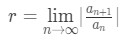Formula 1: Ratio test

Where:

If r < 1, then the series is convergent. A converging series means that the infinite series has a finite sum.

If r > 1 (including infinity), then the series is divergent. This means the infinite series sums up to infinity.

If r = 1, then the series could either be divergent or convergent.

Basically if r = 1, then the ratio test fails and would require a different test to determine the convergence or divergence of the series. I am not going to provide a proof as to why the ratio test works, but this link here provides a step by step formal proof of it.

http://blogs.ubc.ca/infiniteseriesmodule/appendices/proof-of-the-ratio-test/proof-of-the-ratio-test/

Why don't we take a look at some ratio test examples and learn how to fully utilize the ratio test.

## Ratio test for convergence

Let's take a look at the following series:

•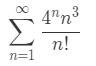Equation 1: Convergence Ratio test pt. 1
• Let's see whether the series converges or diverges. Right away, you see a factorial in the series. This means that ratio test is recommended. Let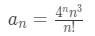Equation 1: Convergence Ratio test pt. 2
• So then we will also have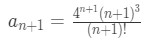Equation 1: Convergence Ratio test pt. 3
• Using these two and plugging them into the ratio test formula will give us: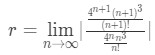Equation 1: Convergence Ratio test pt. 4
• We know that when you are dividing a fraction, then that is the same as multiplying the reciprocal of the fraction. Thus, we can change this to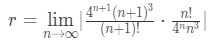Equation 1: Convergence Ratio test pt. 5
• What I like to do is match all the numerators and denominators I see here. Notice that I can match the powers, factorials and polynomials so that it looks like this: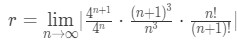Equation 1: Convergence Ratio test pt. 6
• Notice that the powers from the 4's can cancel. Hence this will give us: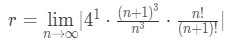Equation 1: Convergence Ratio test pt. 7
• Also, the cube of the polynomials can be factored. Doing so leads to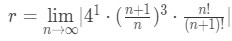Equation 1: Convergence Ratio test pt. 8
• In addition, we can use the factorial rules to expand out the factorials. Doing all of this will give us: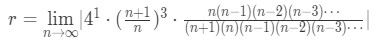Equation 1: Convergence Ratio test pt. 9
• Notice that expanding the factorials leads all the terms to cancel except or n+1 in the denominator. Hence, we can conclude that simplifying factorials leads to: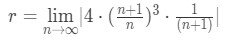Equation 1: Convergence Ratio test pt. 10
• Within the brackets, we are going to divide the n+1 by n, and so we will end up with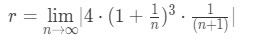Equation 1: Convergence Ratio test pt. 11
• Now we are actually going to take the limit here. Notice that as $n$$\infty$, $\frac{1}{(n+1)}$ goes to 0. In addition, The $1+\frac{1}{n}$ inside the brackets will become 1. Nevertheless, it will be multiplied by 0, so the entire limit will go to 0. Therefore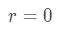Equation 1: Convergence Ratio test pt. 12

We know that 0 < 1, so what does the ratio test conclude? It concludes that the series converge. Note that using the ratio test does not tell you the sum of the series; you would have to use some other means to do that.Now let's take a look at a case in which the ratio test says the series diverges.

## Ratio test for divergence

Let's test for divergence in the following series:

•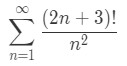Equation 2: Divergence Ratio test pt. 1
• Again we see the factorial, so it will be wise to use the ratio test.

Let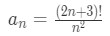Equation 2: Divergence Ratio test pt. 2
• So we will have that: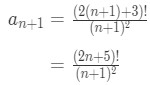Equation 2: Divergence Ratio test pt. 3
• Hence using the ratio test formula will give us: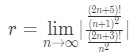Equation 2: Divergence Ratio test pt. 4
• Again we can simply this so that we don't have a fraction on top of a fraction. Instead we can change this to multiplying by the reciprocal of the fraction. This gives us: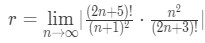Equation 2: Divergence Ratio test pt. 5
• Let's match the polynomials and the factorials so that we have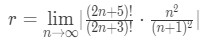Equation 2: Divergence Ratio test pt. 6
• We can expand out the factorials so that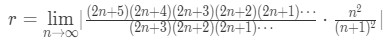Equation 2: Divergence Ratio test pt. 7
• Notice that the terms can cancel so that we can simply the equation to: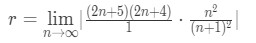Equation 2: Divergence Ratio test pt. 8
• Multiplying the fractions together and expanding the denominator will give us: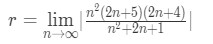Equation 2: Divergence Ratio test pt. 9
• Factoring $n^{2}$ out of the denominator leads to: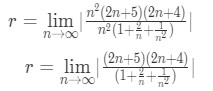Equation 2: Divergence Ratio test pt. 10
• Now we should be able to take the limit as $n$$\infty$. Notice that taking the limit leads to the denominator becoming 1, and the numerator goes to infinity. Hence the entire limit goes to infinity. Hence we can conclude that: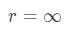Equation 2: Divergence Ratio test pt. 11
• Since $\infty$ > 1, then we can conclude that the series goes to infinity.

If you want to look at more examples of ratio tests with converging or diverging series, click the lesson tab. While we are talking about ratio test, we might as well talk about the root test as well. Why? Because they are very similar!

## Root test

Let there be a series $\sigma a_{n}$. Then we say that: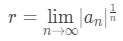Formula 2: Root test

Where:

If r < 1, then the series is convergent

If r > 1 (including infinity), then the series is divergent

If r = 1, then the series could either be divergent or convergent.

Basically if r=1, then the root test fails and would require a different test to determine the convergence or divergence of the series. Realize that these rules are exactly the same as the ratio test! Again, I am not going to provide a proof that the root test works, but I will post a link here showing the proof:

http://people.sju.edu/~pklingsb/pfroot.pdf

Let's look at an example of using the root test!

Consider the following series:

•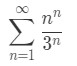Equation 3: Divergence Root test pt. 1
• First we set: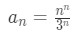Equation 3: Divergence Root test pt. 2
• Now using the root test, we will have that: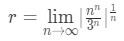Equation 3: Divergence Root test pt. 3
• Notice that we can factor the power of n in both the denominator and numerator so that: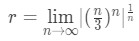Equation 3: Divergence Root test pt. 4
• We can even factor the power of n out of the absolute value so that: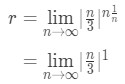Equation 3: Divergence Root test pt. 5
• Now taking the limit of this as $n$$\infty$ will result in r being infinity. In other words,Equation 3: Divergence Root test pt. 6
• Since r = $\infty$ >1, then we know that the series is divergent.

Since we very familiar with the ratio test and root test now, let us talk about finding the interval of convergence.

## How to find interval of convergence

We are able to find the interval of convergence of a power series. Power series are in the form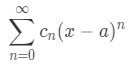Formula 3: Power Series

Where $c_{n}$ are the coefficients of each term in the series and a is a number the series is centred around. We are able to find the radius and interval of convergence if we take either the ratio test or the root test. Depending on the power series, one test may be more convenient than the other. How do we find the interval of convergence using the ratio test?

Let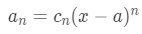Formula 4: Interval of Convergence pt. 1

Then applying the ratio test will give: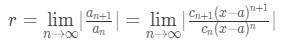Formula 4: Interval of Convergence pt. 2

After taking the limit, set r < 1 and then manipulate the inequality so that it takes the form of |x-a| < R, where R is the radius of convergence.

The interval of convergence is the value of all x's, for which the power series converges. This interval will be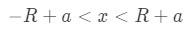Formula 4: Interval of Convergence pt. 3

It is also important to check the endpoints of this inequality (-R+a and R+a) to see if they converge as well. If it is, then include them into the inequality!

Note that there are some cases in which we cannot get the inequality |x-a| < R. You might just end up getting r = 0 < 1. This means that for all x values, r = 0. Hence, the radius of convergence is $\infty$ and the interval of convergence is -$\infty$ < x < $\infty$. You also might get a case where r = $\infty$. In that case, the power series is always divergent for all x.

Alright, now that we know what the radius and interval of converge is, why don't we do a few examples?

Let's take a look at the power series:

•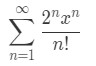Equation 4: Ratio test Interval of Convergence pt. 1
• Since we see factorials, it will be more convenient to use the ratio test here. Let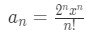Equation 4: Ratio test Interval of Convergence pt. 2
• Then,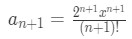Equation 4: Ratio test Interval of Convergence pt. 3
• Applying the ratio test formula gives us: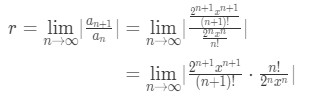Equation 4: Ratio test Interval of Convergence pt. 4
• Matching the powers and the factorials will give us: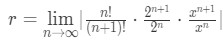Equation 4: Ratio test Interval of Convergence pt. 5
• See here that we can cancel some of the powers so that: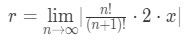Equation 4: Ratio test Interval of Convergence pt. 6
• Expanding the factorials out will lead to lots of terms cancelling: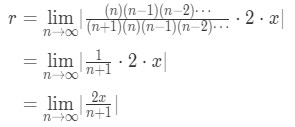Equation 4: Ratio test Interval of Convergence pt. 7
• We can factor x out of the absolute value so that: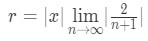Equation 4: Ratio test Interval of Convergence pt. 8
• Notice that we have to put an absolute value around the x when factoring it out of the absolute value. Now taking the limit will give us: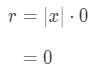Equation 4: Ratio test Interval of Convergence pt. 9

You may see that this is not in the form |x-a| < R. However notice that r = 0 < 1 for all x values. This means that for all x values, the power series converges. Hence the radius of convergence is infinity, and the interval of convergence is -$\infty$ < x < $\infty$ (because it converges everywhere).

How do we find the interval of convergence using the root test? Well again, we just use the root test formula and set r<1and try to get the inequality |x-a| interval of convergence will be. Don't forget to check the endpoints to see if the series is convergent or divergent. If it is convergent, then include it in the interval.

Notice again that you will encounter cases where you will end up either getting r = 0 < 1 or r = $\infty$. r = 0 implies the power series is convergent for all x values, and r = $\infty$ implies the power series is divergent always.

Now let us take a look at a case where we find the interval of convergence using the root test. Consider the power series:

•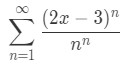Equation 5: Root test Interval of Convergence pt. 1
• We want to use root test here because we see a lot of powers of n. So let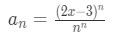Equation 5: Root test Interval of Convergence pt. 2
• Recall that the root test formula isEquation 5: Root test Interval of Convergence pt. 3
• Hence we will get: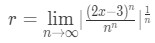Equation 5: Root test Interval of Convergence pt. 4
• Factoring the powers of n out in the absolute value gives: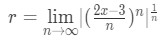Equation 5: Root test Interval of Convergence pt. 5
• Notice that we can factor the power of n outside of the absolute value so that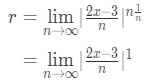Equation 5: Root test Interval of Convergence pt. 6
• We can factor 2x-3 out of the absolute value so that: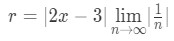Equation 5: Root test Interval of Convergence pt. 7
• Taking the limit will yield the result: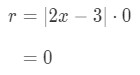Equation 5: Root test Interval of Convergence pt. 8

Again we have the case that r = 0 < 1, hence we can conclude that the power series converge for all x values. In other words, the radius of convergence is $\infty$, and the interval of convergence is -$\infty$ < x < $\infty$.

### Ratio test

In this lesson, we will learn about the ratio test. This test requires you to calculate the value of R using the formula below. If R is greater than 1, then the series is divergent. If R is less than 1, then the series is convergent. If R is equal to 1, then the test fails and you would have to use another test to show the convergence or divergence of the series. It is really recommended to use this test if your series has factorials in it. We will do a few questions which involve using the ratio test, and then look at a question that requires us to use the ratio test twice.

#### Lessons

Note *Let $\sum a_n$ be a series. Then we say that

$R=$$\lim$n →$\infty$ $\mid \frac{a_{n+1}}{a_n}\mid$

Where:
1. If $R$ < $1$, then the series is convergent (or absolutely convergent)
2. If $R$ > $1$, then the series is divergent
3. If $R=1$, then the series could either be divergent, or convergent

Basically if $R=1$, then the ratio test fails and would require a different test to determine the convergence or divergence of the series.
• Introduction
Ratio Test Overview

• 1.
Convergence & Divergence of Ratio Test
Use the Ratio Test to determine if the series converges or diverges. If the ratio test does not determine the convergence or divergence of the series, then resort to another test.
a)
$\sum_{n=1}^{\infty}\frac{4^nn^3}{n!}$

b)
$\sum_{n=0}^{\infty}\frac{(-2)^{2n}}{3^n(n+2)!}$

c)
$\sum_{n=1}^{\infty}\frac{(2n+3)!}{n^2}$

d)
$\sum_{n=0}^{\infty}\frac{3n+6}{n+2}$

• 2.
Using the Ratio Test Twice to Show Convergence
Determine if the series $\sum_{k=1}^{\infty}\frac{4^k+k}{(k+1)!}$ converges or diverges.